### Day 57 - Adding/Subtracting Rational Expressions - 11.10.14

 UpdatesN/ABell RingerPolynomial Division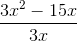x + 5x - 55 - x5 + xnone of the above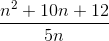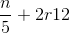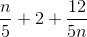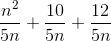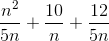none of the above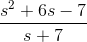s + 7s - 1s + 1s - 7none of the above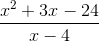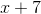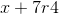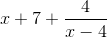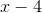none of the above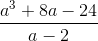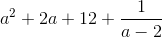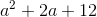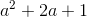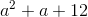ReviewPrerequisitesDividing by 0Rational NumbersNumerator and DenominatorPolynomialsRational FunctionsPolynomial / PolynomialExcluded ValuesGraphs at Excluded ValuesSimplifying Rational ExpressionsMultiplying/Dividing Rational ExpressionsUnit Conversions (Dimensional Analysis)Dividing PolynomialsLong Division of PolynomialsLessonExit TicketPosted on board at end of block. Lesson Objective(s)How can rational expressions be added or subtracted?Standard(s)Solving Rational Equations - 12.9CC.9-12.A.CED.1 Create equations that describe numbers or relationship. Create equations and inequalities in one variable and use them to solve problems. Include equations arising from linear and quadratic functions, and simple rational and exponential functions.CC.9-12.A.REI.11 Represent and solve equations and inequalities graphically. Explain why the x-coordinates of the points where the graphs of the equations y = f(x) and y = g(x) intersect are the solutions of the equation f(x) = g(x); find the solutions approximately, e.g., using technology to graph the functions, make tables of values, or find successive approximations. Include cases where f(x) and/or g(x) are linear, polynomial, rational, absolute value, exponential, and logarithmic functions.CC.9-12.A.REI.2 Understand solving equations as a process of reasoning and explain the reasoning. Solve simple rational and radical equations in one variable, and give examples showing how extraneous solutions may arise.Dividing Polynomials - 12.5CC.9-12.A.APR.6 Rewrite rational expressions. Rewrite simple rational expressions in different forms; write a(x)/b(x) in the form q(x) + r(x)/b(x), where a(x), b(x), q(x), and r(x) are polynomials with the degree of r(x) less than the degree of b(x), using inspection, long division, or, for the more complicated examples, a computer algebra system.Mathematical Practice(s)#1 - Make sense of problems and persevere in solving them#2 - Reason abstractly and quantitatively#4 - Model with mathematics#5 - Use appropriate tools strategically#7 - Look for and make use of structurePast CheckpointsRational ExpressionsCheckpointsSimplifying Rational ExpressionsCheckpointsMultiplying/Dividing Rational ExpressionsCheckpointsDividing PolynomialsCheckpoints